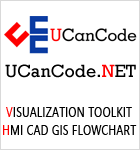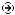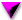YOU CAN CODE!With The Case Of UCanCode.net  Release The Power OF  Visual C++ ! Home |  Products | Purchase |  Support | DownloadsDownload EvaluationPricing & Purchase?E-XD++Visual C++/ MFC ProductsOverviewElectronic Form SolutionVisualization & HMI SolutionPower system HMI SolutionBar code labeling SolutionWorkflow SolutionCoal industry HMI SolutionInstrumentation Gauge SolutionReport Printing SolutionGraphical modeling SolutionGIS mapping solutionVisio graphics solutionIndustrial monitoring SolutionFlowchart and diagramming SolutionOrganization Diagram SolutionGraphic editor Source CodeUML drawing editor Source CodeMap Diagramming SolutionArchitectural Graphic Drawing SolutionRequest EvaluationActiveX COM ProductsOverviewTechnical Support# Get Ready to Unleash the Power of UCanCode .NET

UCanCode Software focuses on general application software development. We provide complete solution for developers. No matter you want to develop a simple database workflow application, or an large flow/diagram based system, our product will provide a complete solution for you. Our product had been used by hundreds of top companies around the world!

"100% source code provided! Free you from not daring to use components because of unable to master the key technology of components!"

 GDI Topics: PolyPolygon, Polyline, Polygon

 Introduction
 The polylines we have used so far were drawn by defining the starting point of the first line and the end point of the last line and there was no relationship or connection between these two extreme points. A polygon is a closed polyline. In other words, it is a polyline defined so that the end point of the last line is connected to the start point of the first line. To draw a polygon, you can use the CDC::Polygon() method. Its syntax is: `BOOL Polygon(LPPOINT lpPoints, int nCount);`

This member function uses the same types of arguments as the Polyline() method. The only difference is on the drawing of the line combination. Here is an example:

 ```void CExoView::OnDraw(CDC* pDC) { CPoint Pt; Pt = CPoint(20, 50); Pt = CPoint(180, 50); Pt = CPoint(180, 20); Pt = CPoint(230, 70); Pt = CPoint(180, 120); Pt = CPoint(180, 90); Pt = CPoint(20, 90); pDC->Polygon(Pt, 7); }```Practical Learning:  Drawing Polygons
1. To draw some polygons, change the program as follows:

 ```void CView1View::OnPaint() { CPaintDC dc(this); // device context for painting // TODO: Add your message handler code here CPoint PtLine[] = { CPoint( 50, 50), CPoint(670, 50), CPoint(670, 310), CPoint(490, 310), CPoint(490, 390), CPoint(220, 390), CPoint(220, 310), CPoint( 50, 310), CPoint( 50, 50) }; CPoint Bedroom1[] = { CPoint( 55, 55), CPoint(175, 55), CPoint(175, 145), CPoint( 55, 145)}; CPoint Closets[] = { CPoint( 55, 150), CPoint(145, 150), CPoint(145, 205), CPoint( 55, 205) }; CPoint Bedroom2[] = { CPoint(55, 210), CPoint(160, 210), CPoint(160, 305), CPoint(55, 305) }; dc.MoveTo(PtLine); dc.LineTo(PtLine); dc.LineTo(PtLine); dc.LineTo(PtLine); dc.LineTo(PtLine); dc.LineTo(PtLine); dc.LineTo(PtLine); dc.LineTo(PtLine); dc.LineTo(PtLine); dc.Polygon(Bedroom1, 4); dc.Polygon(Closets, 4); dc.Polygon(Bedroom2, 4); // Do not call CView::OnPaint() for painting messages }```
 Multiple Polygons

If you want to draw multiple polygons, you can use the CDC::PolyPolygon() method whose syntax is:

`BOOL PolyPolygon(LPPOINT lpPoints, LPINT lpPolyCounts, int nCount);`

Like the Polygon() method, the lpPoints argument is an array of POINT or CPoint values. The PolyPolygon() method needs to know the number of polygons you would be drawing.  Each polygon uses the points of the lpPoints values but when creating the array of points, the values must be incremental. This means that PolyPolygon() will not randomly access the values of lpPoints. Each polygon has its own set of points.

Unlike Polygon(), the nCount argument of PolyPolygon() is the number of polygons you want to draw and not the number of points.

The lpPolyCounts argument is an array or integers. Each member of this array specifies the number of vertices (lines) that its polygon will have..

Here is an example:

 ```void CExoView::OnDraw(CDC* pDC) { CPoint Pt; int lpPts[] = { 3, 3, 3, 3 }; // Top Triangle Pt = CPoint(125, 10); Pt = CPoint( 95, 70); Pt = CPoint(155, 70); // Left Triangle Pt = CPoint( 80, 80); Pt = CPoint( 20, 110); Pt = CPoint( 80, 140); // Bottom Triangle Pt = CPoint( 95, 155); Pt = CPoint(125, 215); Pt = CPoint(155, 155); // Right Triangle Pt = CPoint(170, 80); Pt = CPoint(170, 140); Pt = CPoint(230, 110); pDC->PolyPolygon(Pt, lpPts, 4); }```